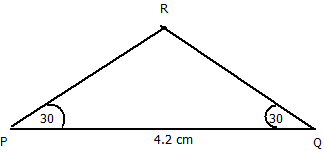### Practical Geometry - Worksheets

CBSE Worksheet-1
CLASS –VII Mathematics (Practical Geometry)

1. Construct an isosceles triangle PQR where the non-equal side PQ = 4.2 cm and base angles are 30° each.
2. If $\mathrm{△}$ABC exactly coincides with $\mathrm{△}$PQR then the triangles are________.
3. In $\mathrm{△}$ABC, BC = CA. Which of its two angles are equal?
4. If AB = QP, AC = QR, BC = PR, then $\mathrm{△}$ABC $\cong$ $\mathrm{△}$QPR, state the congruence criterion involved here.
5. State true or false: The total measure of all the three angles of a triangle is 360°.
6. If we have PQ = 5 cm, $\mathrm{\angle }$PQR= 115° and $\mathrm{\angle }$QRP = 30°, can we construct a $\mathrm{△}$PQR with these measurements?
7. Construct a $\mathrm{△}$LMN, in which MN = 6cm, ML= 4.5 cm and $\mathrm{\angle }$M = 30°.
8. Construct a right triangle PQR in which $\mathrm{\angle }$Q = 90°, PR = 6 cm and QR = 4 cm.
CBSE Worksheet-1
CLASS –VII Mathematics (Practical Geometry)

1.2. congruent.
Explanation:
If three sides and three angles of one triangle are equal to three sides and three angles of second triangle then the two triangles are said to be congruent.
3. $\mathrm{\angle }$A = $\mathrm{\angle }$B.
Explanation:
In an isosceles triangle, the angles opposite to equal sides are equal.
In $\mathrm{△}$ABC, the angle opposite to side BC is $\mathrm{\angle }$A and the angle opposite to side CA is $\mathrm{\angle }$B.
Hence, if BC = CA, then $\mathrm{\angle }$A = $\mathrm{\angle }$B.
4. SSS.
Explanation:
If three sides of a triangle are equal to three corresponding sides of another triangle, then the two triangles are said to be congruent according to SSS congruency criterion.
Given, in $\mathrm{△}$ABC and $\mathrm{△}$QPR,
AB = QP, AC = QR, BC= PR
Therefore, $\mathrm{△}$ABC $\cong$ $\mathrm{△}$QPR , by SSS congruency criterion.
5. False.
Explanation:
According to angle sum property of a triangle, sum of 3 angles of a triangle should be 180°.
6. Yes.
Explanation:
Given, in $\mathrm{△}$PQR, PQ = 5 cm, $\mathrm{\angle }$PQR= 115° and $\mathrm{\angle }$QRP = 30°
We can locate point R, by constructing the third $\mathrm{\angle }$QPR = 35° [180°- (115° + 30°)] from the point P, which meets $\mathrm{\angle }$PQR at R